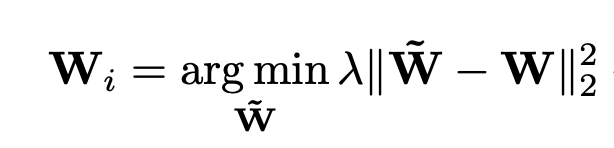# Getting RuntimeError: element 0 of tensors does not require grad and does not have a grad_fn but the element 0 in my case is just a tensor of fixed float values that only needs to be subtracted from nn.Parameter and hence, does not need the grad_fn

Hey!
I’m trying to implement a text classification model that retrieves K batches from replay memory based on nearest neighbour approach(using a specific encoding of our test document that needs to be classified as key) and first trains on this batch to adjust to adjust its weight minimising log likelihood loss. However, an additional constraint is to be enforced which tries to minimise the euclidean distance between the original weight parameters and the newly trained parameters.
Hence, I’m trying to define a custom loss function to enforce the weight constraint:where,
W contains weight parameters to be trained
˜W contains the base parameters
I’ve freezed the base network’s weights since they are not supposed to be changed

``````# base model weights
self.base_weights = list(self.classifier.parameters())
# # Freeze the base model weights
for param in self.base_weights:
``````

And here is my local adaptation code:

``````# create a local copy of the classifier network
# Current model weights
# Train the adaptive classifier for L epochs with the rt_batch
for _ in trange(self.L, desc='Local Adaptation'):

diff = torch.Tensor().cuda()
# Iterate over base_weights and curr_weights and accumulate the euclidean norm
# of their differences
for base_param, curr_param in zip(self.base_weights, curr_weights):
diff += (base_param-curr_param).pow(2).sum()
# Total loss due to log likelihood and weight restraint
diff_loss = 0.001*diff.sqrt()
diff_loss.backward()
likelihood_loss.backward()
optimizer.step()
``````

But when I try to run the code, I get the error
` RuntimeError: element 0 of tensors does not require grad and does not have a grad_fn`
I freezed the base_weights because I got `RuntimeError: Cuda Out of Memory ` when they had not been. I suppose the weights were being tracked causing the above mentioned error.
Can anyone please point out the flaws in my implementation?
Or please suggest a more efficient implementation.
Any help would be highly appreciated.
Thanks in advance1 Like

Hi,

Few things:

• You can replace this to be more efficient:
``````diff_loss.backward()
likelihood_loss.backward()
``````

by

``````(diff_loss + likelihood_loss).backward()
``````
• When you do `list(foo.parameters())`, you get a reference to the weights. So if you change them to not require gradients, then you basically change all your weights in your network not to require gradients. You can add `.clone().detach()` if you want to get a different Tensor that does not require gradients.
1 Like

Thanks.
I thought that model.parameters() returned a copy of weights.
I solved the issue by creating an empty `base_weights` list and appended the individual parameter weight data. However, your method seems more compact and wouldn’t require the cuda() method to be called on the weights

``````  # base model weights
self.base_weights = list()
for param in self.classifier.parameters():
self.base_weights.append(param.data.cuda())
``````

Small detail: You should not do `param.data.cuda()` but `param.detach().cuda()`. As `.data` should not be used anymore.

1 Like